### Home > CALC > Chapter 4 > Lesson 4.4.1 > Problem4-125

4-125.
1. A horizontal flag is shown below. The radius of the outer semicircle is 4, while that of the inner semicircle is 3. Homework Help ✎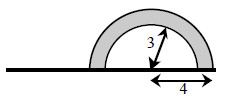1. Imagine rotating the flag about its pole and describe the resulting three-dimensional figure. Draw a picture of this figure on your paper.

2. Find the volume of the rotated flag.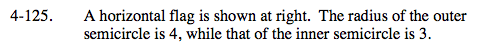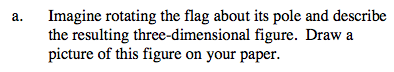Imagine a hollow rubber ball. The rubber is 1-inch thick and the radius is 3 inches.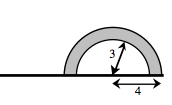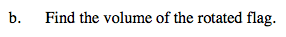Volume = big sphere − small sphere

$\frac{4}{3}\pi(4)^3-\frac{4}{3}\pi(3)^3=\frac{148}{3}\pi \text{ un}^{3}$